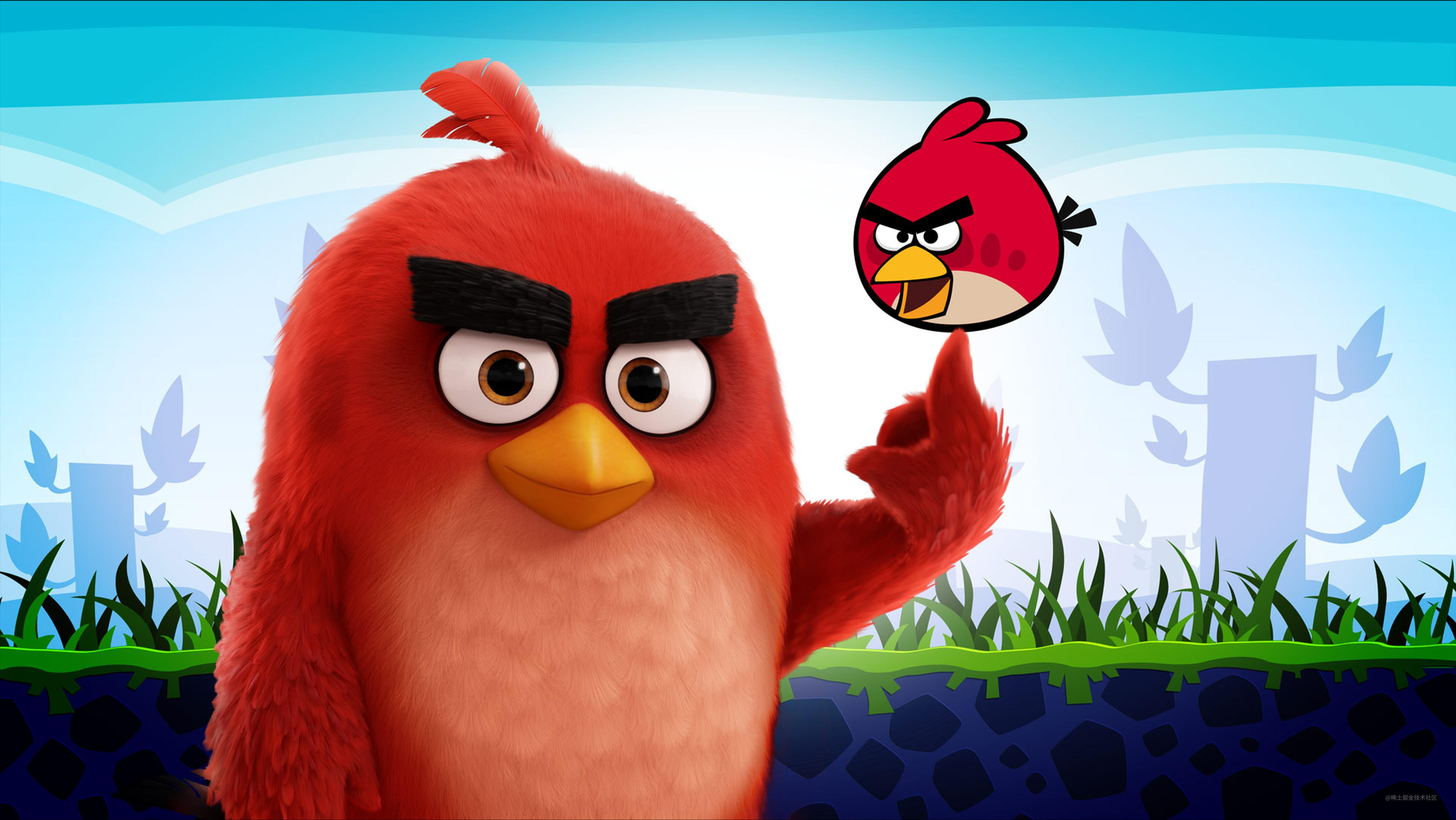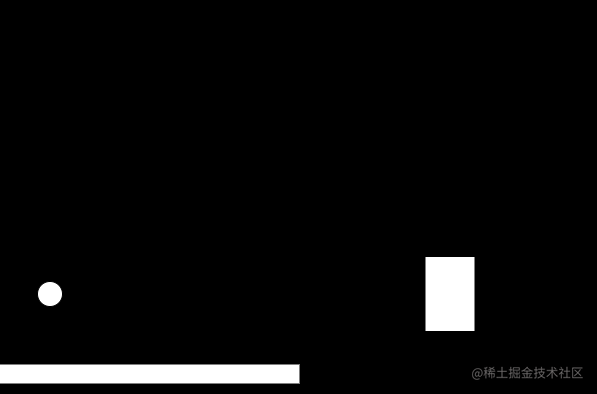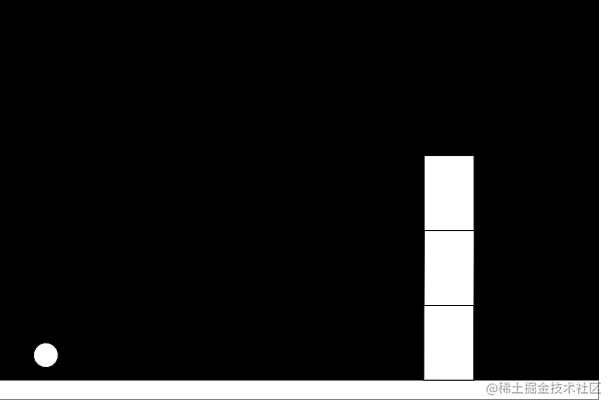# 写一个愤怒的小鸟 (2)「这是我参与11月更文挑战的第12天，活动详情查看：2021最后一次更文挑战

``````show(){
const pos = this.body.position;
const angle = this.body.angle;
push()
translate(pos.x,pos.y)
rotate(angle)
fill(255)
rect(0,0,this.w,this.h)
pop()
}

``````    show(){
...
fill(255);
rectMode(CENTER);
rect(0,0,this.w,this.h);
pop();
}### 启动引擎

``````  function draw() {
background(0);
Matter.Engine.update(engine)
ground.show();
box.show();
bird.show();
}

``````class Ground extends Box{
constructor(x,y,w,h){
super(x,y,w,h);
this.body.isStatic = true;
}
}

### 对 Circle 进行改造

``````class Bird{
constructor(x,y,r){
this.body =Matter.Bodies.circle(x, y, r);
this.r = r;
}

show(){
const pos = this.body.position;
const angle = this.body.angle;
push();
translate(pos.x,pos.y);
rotate(angle);
fill(255);
rectMode(CENTER);
circle(0,0,this.r);
pop();
}
}

• 首先创建一个 `Matter.Bodies` 的几何体作为刚体代理
• 然后将创建刚体代理添加物理引擎的 world
• 然后从刚体上获取位置和角度信息
• 利用角度和位置信息以及几何体大小信息来绘制几何体

#### 添加互动

``````const {
Engine,
World,
Bodies,
Mouse,
MouseConstraint,
Constraint
} = Matter

``````function setup() {
...

const mouse = Mouse.create(canvas.elt);
const options = {
mouse: mouse
}

mConstraint = MouseConstraint.create(engine,options);

}

### 添加多个 box

``````...

let ground;
let boxes = [];

...
function setup() {
...
for (let i = 0; i < 3; i++) {
boxes[i] = new Box(450,300 -i*75,50,75);

}
...

}

function draw() {
...
for (let i = 0; i < 3; i++) {
boxes[i].show();
}
...
}